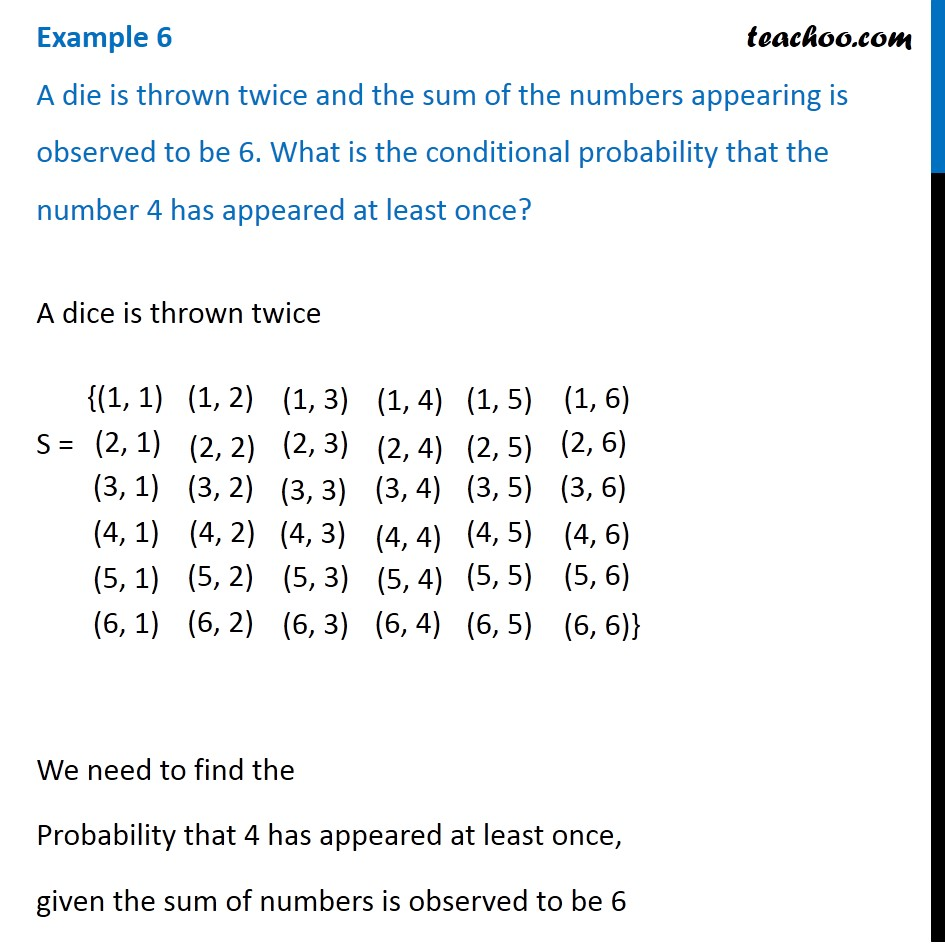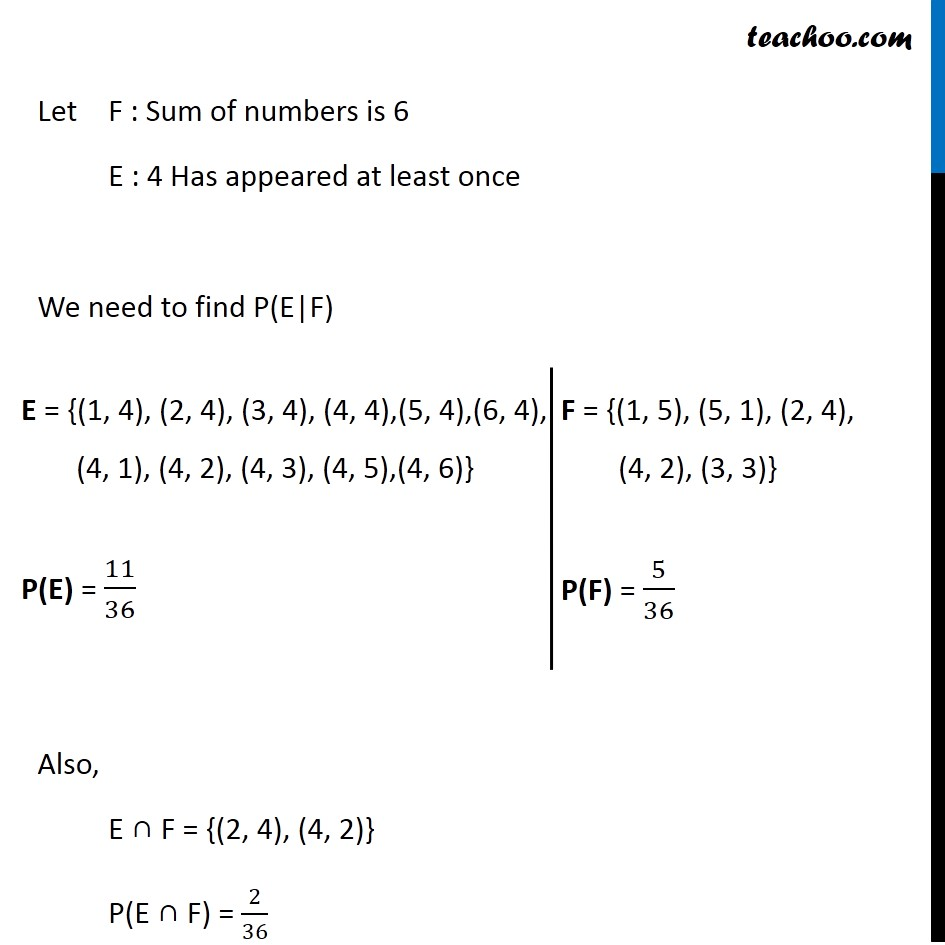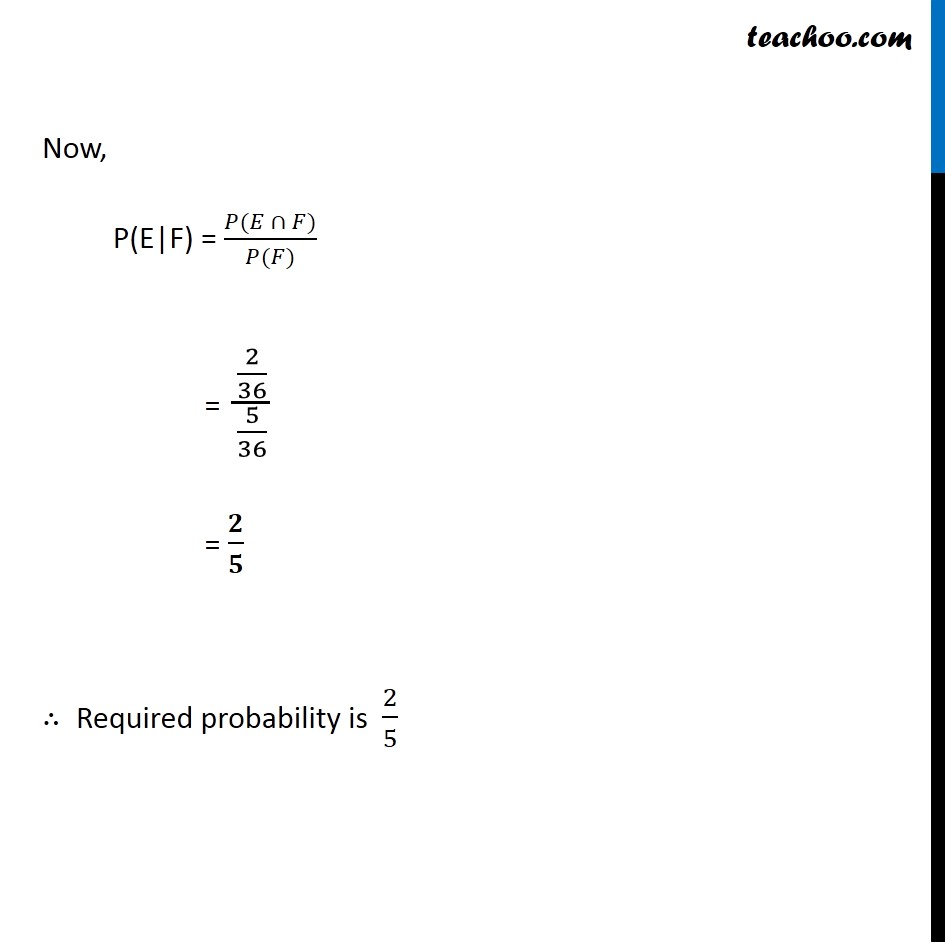Chapter 13 Class 12 Probability

Class 12
Important Questions for exams Class 12Learn in your speed, with individual attention - Teachoo Maths 1-on-1 Class

### Transcript

Example 6 A die is thrown twice and the sum of the numbers appearing is observed to be 6. What is the conditional probability that the number 4 has appeared at least once? A dice is thrown twice S = We need to find the Probability that 4 has appeared at least once, given the sum of numbers is observed to be 6 Let F : Sum of numbers is 6 E : 4 Has appeared at least once We need to find P(E|F) Also, E ∩ F = {(2, 4), (4, 2)} P(E ∩ F) = 2/36 Let F : Sum of numbers is 6 E : 4 Has appeared at least once We need to find P(E|F) Also, E ∩ F = {(2, 4), (4, 2)} P(E ∩ F) = 2/36 E = {(1, 4), (2, 4), (3, 4), (4, 4),(5, 4),(6, 4), (4, 1), (4, 2), (4, 3), (4, 5),(4, 6)} P(E) = 11/36 F = {(1, 5), (5, 1), (2, 4), (4, 2), (3, 3)} P(F) = 5/36 Now, P(E|F) = (𝑃(𝐸 ∩ 𝐹))/(𝑃(𝐹)) = (2/36)/(5/36) = 𝟐/𝟓 ∴ Required probability is 2/5